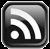# LEARN MS-ACCESS TIPS AND TRICKS

Learn Microsoft Access Advanced Programming Techniques, Tips and Tricks.

### Join Split Array Functions

The above MS-Access functions are not so popular or used frequently in programs, but their usage is very interesting and powerful too. Let us take one by one and learn how powerful they are?  We will write a program later to demonstrate their usage in real world programs.

Let us take the Array() Function first.  Before we try the Array() Function let us find out how to define an array variable and assign values into each element of the array?

```'dimension the array for six elements
Dim varNumber(0 To 5) As Variant, j As Integer
For j = 0 To 5
varNumber(j) = j + 1
Next```
1. The first statement in the above program defines a variable varNumber as a Variant Type array for 6 elements.
2. Another variable j is defined as an integer that will be used as an index variable in the For. . .Next loop.
3. The next three statements in the above program assigns values 1 to 6 into the array elements as:
• varNumber(0) = 1
• varNumber(1) = 2
• varNumber(2) = 3
• varNumber(3) = 4
• varNumber(4) = 5
• varNumber(5) = 6

If we attempt to assign a value into element varNumber(6) then it will run into error because we have not dimensioned the array beyond the varNumber(5) element.

We can do this task with only one statement if we use the Array() Function as below:

varNumber = Array(1,2,3,4,5,6)

We don't have to define the variable for a fixed number of elements as we did in the first statement, it does this task automatically depending on the number of items in the argument list.  We have used constant values 1 to 6 to assign into the array elements.  Another thing to keep in mind is that the target variable varNumber must be always defgined as a Variant Type Variable.  That gives us more flexibility in assigning mixed Data Type values into different elements of the target variable like the example given below:

varNumber = Array("Nancy",25,"Andrew",30,172.5)

We have assigned a mix of String, Integer and Double Data Type values into different elements of the same array.  Again we have used constant values to assign into the array.

This Function is very useful to pass several parameters to a Program as a single block without defining several parameter declarations in the main program.

You can use Constants, Variables or data Field Values to assign values into the array.

Example-1:

``` a = "Nancy"
b = 25
c = 172.5
varNumber = Arrary(a,b,c)
```

Example-2:

``` varNumber = Array(Me![FirstName],Me![BirthDate],Me![Address])
```

Next we will examine the usage of Join() Function. Let us make another array of values for this function so that its usage is understood easily.

``` varNumber = Array("Sun","Mon","Tue","Wed","Thu","Fri","Sat")
```

We have the names of week days in the array variable varNumber.

``` varNumber(0) = "Sun"
.
.
.
varNumber(6) = "Sat"
```

If you want to combine all values from seven elements of this array variable and create a single string; each item separated with commas (like: Sun,Mon,Tue,Wed,Thu,Fri,Sat) then in normal case you must write the following statements to achieve this result:

```Dim strWeeks as string, j as integer
strWeeks=""
For j = 0 to 6
if j=6 then
strWeeks = strWeeks & varNumber(j)
Else
strWeeks = strWeeks & varNumber(j) & ","
end if
Next```

The above task takes only one statement with the Join() Function:

strWeeks = Join(varNumber,",")

The first parameter to the Join() Function is the array of values to be joined together to form a string.  Second parameter is the item separator character; comma, if omitted a space character will be used as separator character by default, otherwise whatever character you specify will be used as separator.

Result: strWeeks = "Sun,Mon,Tue,Wed,Thu,Fri,Sat"

Split() is the complementary Function of Join().  It splits the individual item separated by the delimiter character and stores the values into an array variable of Variant Type.

We need the following lines of code to do the same task of Split() Function:

```Dim strWeeks(0 To 6) As Variant, strtxt As String
Dim j As Integer, k As Integer

strtxt = "Sun,Mon,Tue,Wed,Thu,Fri,Sat"
k = 0
For j = 1 To Len(strtxt) Step 4
strWeeks(k) = Mid(strtxt, j, 3)
k = k + 1
Next```

With the use of Split() Function it takes only one statement to do the job that we did with the above program:

strWeeks = Split(strTxt,",")

Next Week we will use these functions in a Program to redefine a Query linked to a Form to filter and view data.

Share:

#### 1 comment:

1.## TranslateSubscribe in a readerYour email address:

Delivered by FeedBurner

## Featured Post

### Add Class Objects as Dictionary Items

We have learned the fundamentals of Dictionary Object, done trial runs for sorting simple items and displayed Access Table records through D...